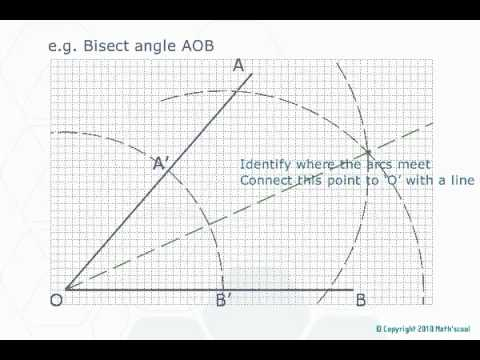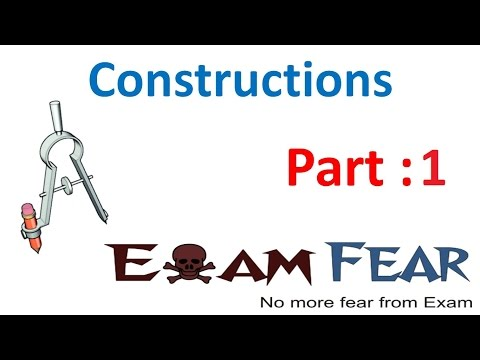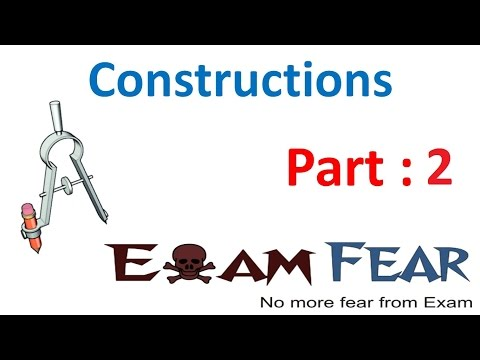## Construction

Subject: Compulsory Maths

#### Overview

A line segment which is perpendicular to a given line segment and bisects the line segment is called the perpendicular bisector of the given line segment. The process of constructing an angle to the given vertex is called transferring angles.

#### Construction of perpendicular bisector of a line segment

A line segment which is perpendicular to a given line segment and bisects the line segment is called the perpendicular bisector of the given line segment.

Draw a line segment AB and construct it perpendicular bisector.

Steps of construction

1. Draw a line segment AB.
2. With centre at A and radius more than half of AB, draw two arcs above and below AB.
3. With centre at B and the same radius, draw two arcs intersecting the first two arcs at P and Q respectively.
4. Join P and Q. PQ intersects AB at C. Measure AC, BC and $\angle$PCA (or $\angle$PCB)
Here, AC = BC and $\angle$PCA = 90°.
$\therefore$ PQ is theperpendicular bisector of AB.

Transferring Angles

The process of constructing an angle to the given vertex is called transferring angles.Construct an angle at Q equal to $\angle$AOB

Steps of construction

1. Fron the point Q, draw a line segment QP.
2. With centre at O and suitable radius draw an arc to intersect OA at C and OB at D.
3. With the centre Q, draw the same arc intersect QP at S.
4. Measure the length of CD with the help of compasses and draw an arc of the same.
5. Join Q and T and produce it to R.
Thus $\angle$PQR = $\angle$AOB is drawn.

#### Construction of different angles

Construct 60°, 120° and 90° with the help of a compass.

Steps of construction

1. Draw a line segment AB
2. With centre at A and a suitable radius, draw an arc to cut AB at P.
3. From P, draw an arc with the same radius to cut the first arc at Q. Join A, Q and produce to C.
Here, $\angle$BAC = 60°
4. From Q draw an arc with the same radius to cut the first arc at R. Join A, R and produce to D.
Here, $\angle$BAD = 120°
5. From Q and R draw two arcs with the same radius to intersect each other at S.
6. Join A and S.
Here, $\angle$BAS = 90°.

#### Construction of triangles

Let's learn to construct the various types of triangles under the following conditions.

When the lengths of 3 sides are given:

Construct a triangle PQR in which PQ = 6 cm,QR = 4 cm and RP = 3.5 cm.

Steps of construction

1. Draw a line segment PQ = 6 cm.
2. With centre at Q and radius 4 cm, draw an arc.
3. With centre P and radius 3.5 cm, draw another arc to intersect the first arc at R.
4. Join P, Rand Q, R.
Thus, PQR is the required triangle.

#### When the lengths of two sides and angle made by them are given

Construct a triangle ABC in which AB = 5 cm, AC = 3 cm and $\angle$A = 45°.

Steps of construction

1. Draw a line segment AB = 5cm.
2. At A, construct $\angle$BAX =45°.
3. With centre at A and radius 3 cm, draw an arc to cut AX at C.
4. Join B and C.
Thus, ABC is the required triangle.

When two angles and their common adjacent sides are given:

Construct a triangle ABC in which

AB = 6 cm, $\angle$A = 60° and $\angle$B = 30°.

Steps of construction

1. Draw a line segment AB = 6 cm
2. AT A and B construct $\angle$BAX = 60° and $\angle$ABY = 30° respectively. AX and BY intersect each other at C.
Thus, ABC is the required triangle.

When lengths of hypotenuse and a side of a right-angled triangle are given:

Construct a right angled triangle ABC in which a side AB = 5.5 cm and hypotenuse AC = 7 cm.

Steps of construction

1. Draw a line segment AB = 5.5 cm
2. At B, construct $\angle$ABX = 90°
3. With centre A and radius 7cm, draw an arc to intersect BX at C.
4. Join A and C.
Thus, ABC is the required right angled triangle.

### Construction of parallelograms

Let's construct parallelograms under the following given conditions.

Construct a parallelogram ABCD in which AB = 5 cm, BC = 4 cm and $\angle$ABC = 60°.

Step of construction

1. Draw a line segment AB = 5 cm.
2. At B, construct $\angle$ABX = 60°.
3. With the centre at B and radius BC = 4 cm, draw an arc to intersect BX at C.
4. With centre at C and radius CD = AB = 5 cm, draw an arc.
5. With centre at A and radius AD = BC = 4 cm, draw another arc to cut the previous arc at D.
6. Join A, D and C, D.
7. Thus, ABCD is the required parallelogram.

When base, diagonal and angle made by the diagonal with base are given:

Construct a parallelogram ABCD in which base AB = 6 cm, diagonal 7 cm and $\angle$BAC = 30°.

Steps of construction

1. Draw base AB= 6 cm
2. At A, construct $\angle$BAX = 30°.
3. With centre at A and radius AC = 7 cm, draw an arc to cut AX at C.
4. With centre at C and radius CD = AB = 6 cm, draw an arc.
5. With centre at A and radius
AD = BC, draw another arc to cut the previous arc at D.
6. Join A, D and B, C.
Thus ABCD is the required parallelogram.

When the length of diagonals and an angle made by them are given:

Construct a parallelogram ABCD in which diagonals AC = 4 cm, BD = 5 cm and they bisect each other making an angle of 30°.

Steps of construction

1. Draw a diagonal AC = 4 cm
2. Draw the perpendicular bisector of AC and mark its mid-point O.
3. At O, construct $\angle$AOX = 30° and produce XO to Y.
4. Here, O is also the midpoint of BD. With centre at O and radius OB = OD = 2cm.
5. Join A, D; B, C; A, B and C, D.
Thus, ABCD is the required parallelograms.

#### Construction of squares

Let us construct squares under the following conditions:

When a side of a square is given

Construct a square ABCD in which AB = 5cm.

Steps of construction

1. Draw a line segment AB = 5 cm.
2. At A and B construct $\angle$BAX = 90° and $\angle$ABY = 90° respectively.
3. With centres at A and B and radius 5 cm, cut AX at D and BY at C.
4. Join C, D.
Thus, ABCD is the required square.

When the length of a diagonal is given

Construct a square ABCD in which diagonal BD = 4 cm.

Steps of construction:

1. Draw a diagonal BD = 4 cm.
2. Diagonals bisect the angles of a square.
So, construct $\angle$DBX = $\angle$BDY = 45°.
3. Let, BX and DY intersect at A.
4. With centres at B and D and radius equal to AB (or AD), draw two arcs intersecting at C.
5. Join B, C and D, C.
Thus, ABCD is the required square.

#### Construction of rectangles

When two adjacent sides of a rectangle are given:

Construct a rectangle ABCD in which AB = 5cm and BC = 4cm.

Steps of construction:

1. Draw a line segment AB = 5 cm.
2. At A and B, construct $\angle$ABX = $\angle$BAY = 90°.
3. With the centres A and B and radius 4 cm, draw two arcs to cut AY at D and BX at C.
4. Join C and D.
Thus, ABCD is the required rectangle.

When the diagonals and an angle made by them are given:

Construct a rectangle ABCD in which diagonal AC = BD = 6 cm and they bisect each other making 60° angles.

Steps of construction:

1. Draw AC= 6 cm and draw its perpendicular bisector to find its mid-point O.
2. At O, construct $\angle$AOX = 60° and produce XO to Y.
3. With centre at O and radius 3cm, draw two arcs to cut OX at D and OY at B.
4. Join A, D; B, C;A, B and C, D.
Thus, ABCD is the required rectangle.

#### Construction of rhombus

When a side and angle made by two adjacent sides are given:

Construct a rhombus ABCD in which AB = 6 cm and $\angle$ABC = 45°.

Steps of construction

1. Draw AB = 6cm.
2. At B, construct $\angle$ABX = 45°.
3. With centre at B and radius 6 cm, cut BX at C.
4. With centres at A and C and radius 6 cm, draw two arcs intersecting each other at D.
5. Join A, D and C, D.
Thus, ABCD is the required rhombus.

When two diagonal are given

Construct a rhombus ABCD in which diagonals AC = 5 cm and BD = 3cm.

Steps of construction

1. Draw AC = 5 cm and draw its perpendicular bisector XY. Mark the mid-point of AC as O.
2. With centre at O and radius 2 cm, draw two arcs to cut OX at D and OY at B.
3. Join A, B; B, C; C, D and D, A.
Thus ABCD is the required rhombus.

#### Construction of kite

When two unequal adjacent sides and angle between them are given:

Construct a kite ABCD in which AB = 5 cm AD = 3 cm and $\angle$BAD = 120°.

Steps of construction

1. Draw AB = 5 cm.
2. At A, construct $\agle$BAX = 120°.
3. With centre at D and radius 3 cm, cut AX at D.
4. With centre at D and radius 3 cm, draw an arc. With centre at B and radius 5 cm, draw another arc to cut the previous arc at C.
5. Join C, D and B, C.
Thus, ABCD is the required kite.

When two unequal adjacent sides and angle between two equal adjacent sides are given:

Construct a kite ABCD in wich AB = 4 cm, BC = 6 cm and $\angle$BAD = 90°.

Steps of construction

1. Draw AB = 4 cm.
2. At A, construct $\angle$BAX = 90°.
3. With centre at A and radius 4 cm, draw an arc to cut AX at D.
4. With centres at D and B and B and radius 6 cm, draw two arcs intersecting each other at C.
5. Join C, D and B, C.
Thus, ABCD is the required kite.

When two diagonals are given:

Construct a kite ABCD in which diagonals AC = 6 cm andBD = 5 cm.

Steps of construction

1. Draw AC = 6 cm.
2. Mark any point ) on AC and construct $\angle$AOX = 90°. Produce XO to Y.
3. With centre at O and radius 2 cm, draw two arcs to cut OX at D and OY at B.
4. Join A, B; A, D; B, C and C, D.
Thus, ABCD is the required kite.

##### Things to remember
• A line segment which is perpendicular to a given line segment and bisects the line segment is called the perpendicular bisector of the given line segment.
• The process of constructing an angle to the given vertex is called transferring angles.
• It includes every relationship which established among the people.
• There can be more than one community in a society. Community smaller than society.
• It is a network of social relationships which cannot see or touched.
• common interests and common objectives are not necessary for society.
##### Videos for Construction##### Maths :|: Constructions and Loci :|##### Maths Constructions part 1 (Divide a line in a ratio)##### Maths Constructions part 2 (Divide a triangle in a ratio)

Solution:

1. Draw a line segment AB
2. With centre at A and a suitable radius, draw an arc to cut AB at P.
3. From P, draw an arc with the same radius to cut the first arc at Q. Join A, Q and produce to C.
Here, $\angle$BAC = 60°
4. From Q draw an arc with the same radius to cut the first arc at R. Join A, R and produce to D.
Here, $\angle$BAD = 120°
5. From Q and R draw two arcs with the same radius to intersect each other at S.
6. Join A and S.
Here, $\angle$BAS = 90°.

Solution:

1. Draw a line segment PQ = 6 cm.
2. With centre at Q and radius 4 cm, draw an arc.
3. With centre P and radius 3.5 cm, draw another arc to intersect the first arc at R.
4. Join P, Rand Q, R.
Thus, PQR is the required triangle.

Solution:

1. Draw a line segment AB = 5cm.
2. At A, construct $\angle$BAX =45°.
3. With centre at A and radius 3 cm, draw an arc to cut AX at C.
4. Join B and C.
Thus, ABC is the required triangle.

Solution:

1. Draw a line segment AB = 5 cm.
2. At B, construct $\angle$ABX = 60°.
3. With the centre at B and radius BC = 4 cm, draw an arc to intersect BX at C.
4. With centre at C and radius CD = AB = 5 cm, draw an arc.
5. With centre at A and radius AD = BC = 4 c, draw another arc to cut the previous arc at D.
6. Join A, D and C, D.
7. Thus, ABCD is the required parallelogram.

Solution:

1. Draw base AB= 6 cm
2. At A, construct $\angle$BAX = 30°.
3. With centre at A and radius AC = 7 cm, draw an arc to cut AX at C.
4. With centre at C and radius CD = AB = 6 cm, draw an arc.
5. With centre at A and radius
AD = BC, draw another arc to cut the previous arc at D.
6. Join A, D and B, C.
Thus ABCD is the required parallelogram.

Solution:

1. Draw a line segment AB = 5 cm.
2. At A and B construct $\angle$BAX = 90° and $\angle$ABY = 90° respectively.
3. With centres at A and B and radius 5 cm, cut AX at D and BY at C.
4. Join C, D.
Thus, ABCD is the required square.

Solution:

1. Draw AC= 6 cm and draw its perpendicular bisector to find its mid-point O.
2. At O, construct $\angle$AOX = 60° and produce XO to Y.
3. With centre at O and radius 3cm, draw two arcs to cut OX at D and OY at B.
4. Join A, D; B, C;A, B and C, D.
Thus, ABCD is the required rectangle.

Solution:

1. Draw AB = 6cm.
2. At B, construct $\angle$ABX = 45°.
3. With centre at B and radius 6 cm, cut BX at C.
4. With centres at A and C and radius 6 cm, draw two arcs intersecting each other at D.
5. Join A, D and C, D.
Thus, ABCD is the required rhombus.

Solution:

2. At A, construct $\agle$BAX = 120°.